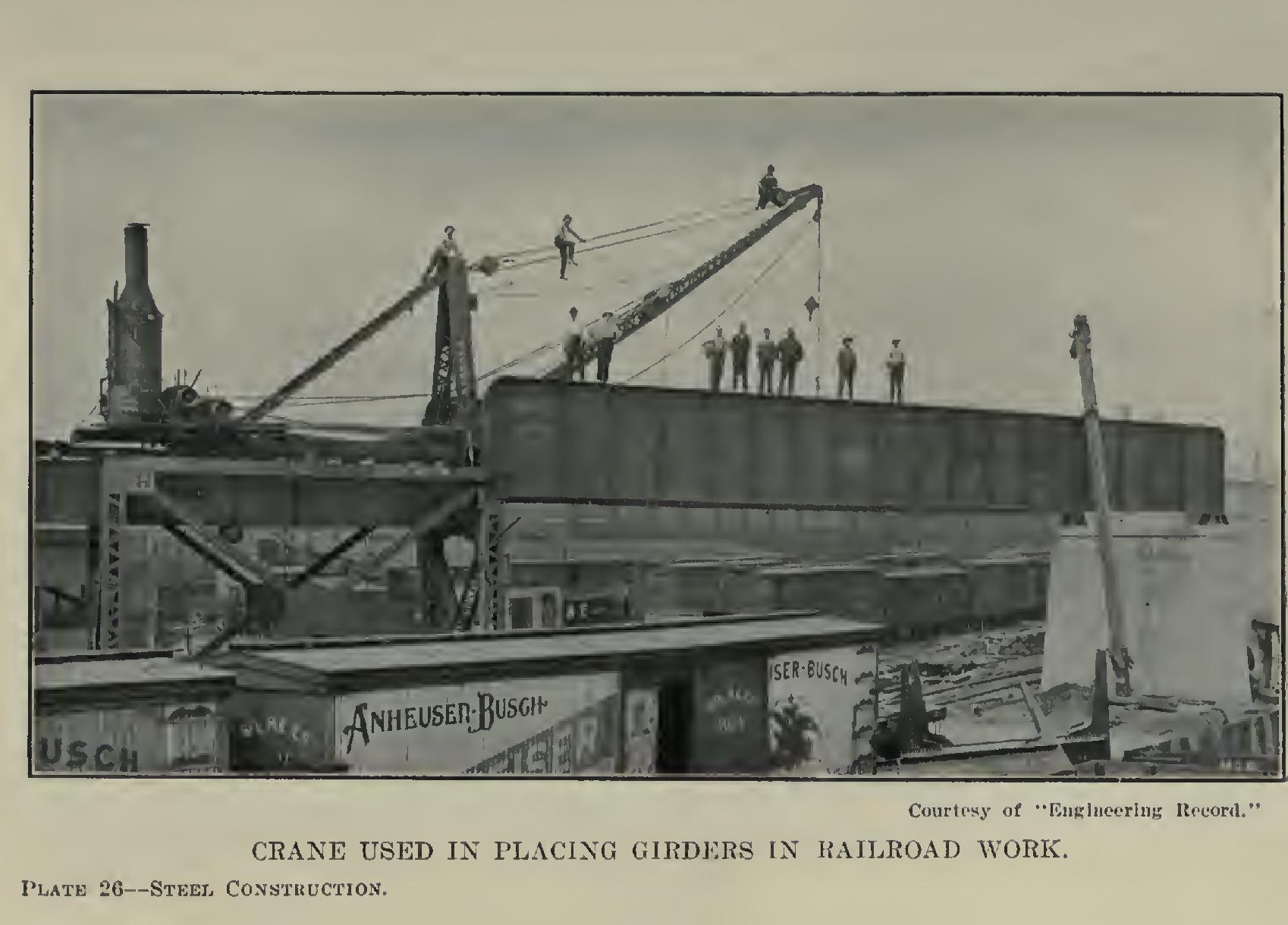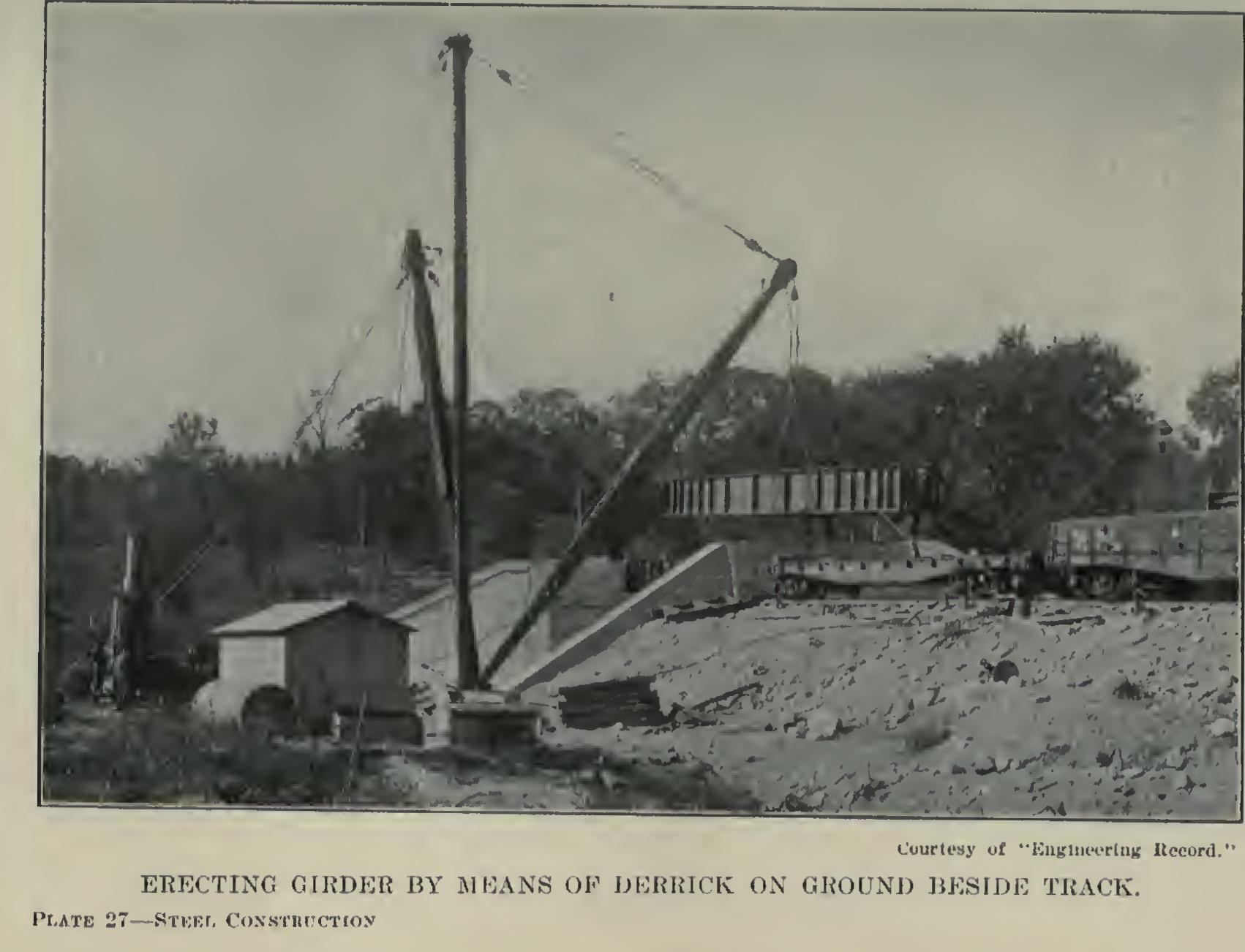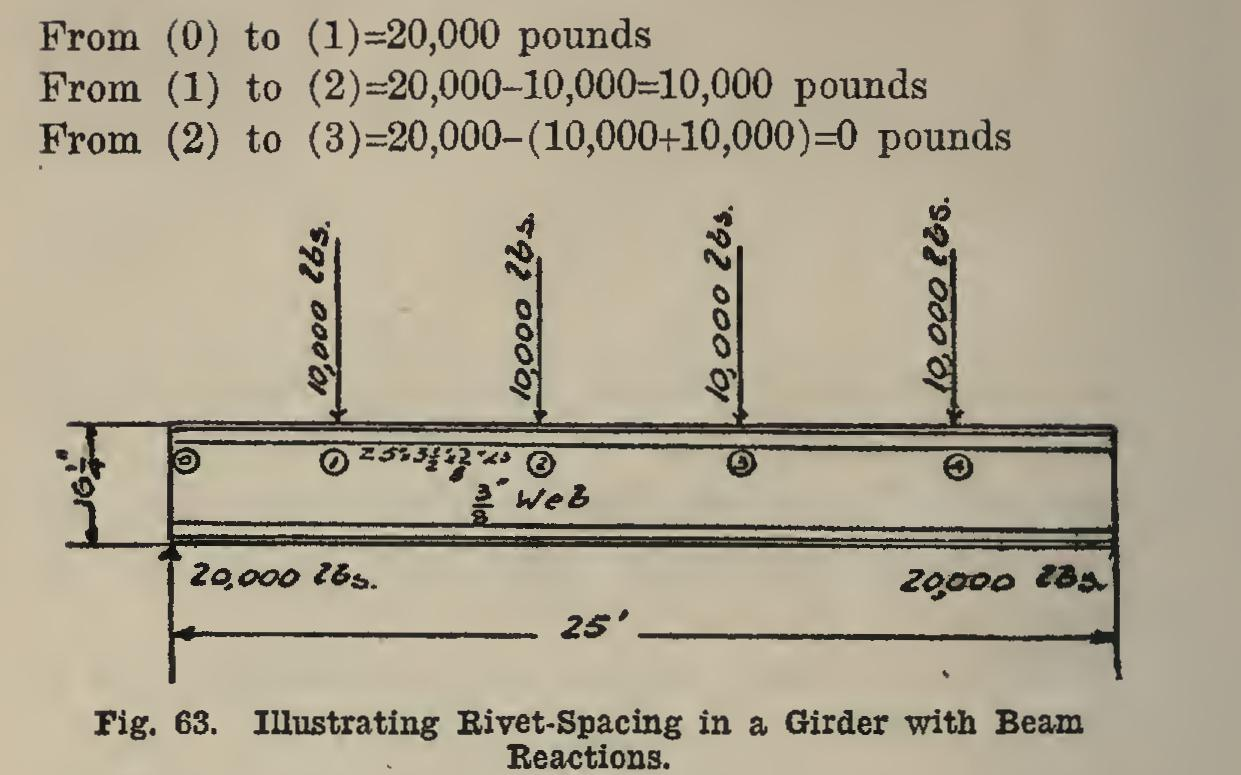# Girders 40

## rivets, inches, spacing, angle, girder, shear and rivet

Page: 1 2 3 4 5 6 7

More field than shop rivets are required to hold the same force, since it is supposed that a rivet driven by pneumatic or hand tools is not so strong as one driven by the large power riveters in the shops. Fig. 62 shows the connection and where the rivets should go. Field rivets are shown, as such connections are usually field con nections.The rivets as above computed are those which go through the column and the connection an gles of the girder. The number of rivets which go through the girder itself and the connection angles must also be determined. Since all webs are % inch thick, except in rare cases, this will be the only case treated.

The number of shop rivets is equal to the shear at that end (that is, the end reaction), di vided by the value of a rivet in single shear. This riveting is seldom made other than in the shop; but, in case it is done in the field, the field rivets are determined as before. In the case just treated, the required number is 87,500-i-6,010=15 (shop) ;15 X field.

Fig. 62 shows the location, number, and character of these rivets.

In case all the rivets cannot be placed in one row without crowding the spacing too much, the angle should have a leg wide enough to let them be staggered in two rows. The flanges must be riveted to the web.

Rivet-Spacing in Girder Flanges. The rivet spacing depends upon the shear, upon the thick ness of the web, upon the value of a rivet, and upon the distance between the rivet-lines of the two flanges.

The last condition depends upon the distance of the rivets from the back of the angles. All rivets are spaced at a certain distance from the back of the angles, and this distance is standard for a given size of angle. The distance is called the gauge of the angle. When an angle can have two lines of rivets in it, that angle is said to have a double gauge. The line on which they are placed is called the gauge-line. • In double gauges, the second gauge-line is measured from the first one, instead of from the back of the angle. Table XIV gives the standard gauges for any sized angle leg.In all flanges except those which have a uni form load distributed over them (for example, a girder which has a railroad track running over the top), the rivet-spacing at any point is: This means that the spacing is equal to the value of a rivet multiplied by the distance between gauge-lines, and the result is then divided by the shear at that point. All dimensions are in

inches.

In cases where beams rest upon the top of a girder, stiffeners should be placed at that point.

In case of railroad girders, as described above, compute the spacing by the given form ula, and reduce it 1/2 inch. This is not the tech nically exact method; but since it is on the safe side, and the exact method of calculation involve complicated computations in higher mathematics, the use of this formula will be found perfectly practicable.

In the above formula, v is the value of a rivet in bearing in the web of the girder for all thicknesses of web up to inch inclusive; in webs thicker than inch, it is the double shearing value of the rivet.

In Fig. 63 is shown a girder with reactions of beams at four places along its length. If the third table (C 195) is used, what will be the rivet-spacing'? The shears are computed, and are: The value of v, the web being is 6,570 poimds. We compute h (see Table XIV) as fol lows: X inches. Therefore, 6,570x12f From (0) to (1), s= 20,000 —3.9, say 3 inches.6,570x121 From (1) to (2), s= 10,000 =7.8, say 7/ inches.

6,570x12/ From (2) to (3), s= 0 =as large as imaginable.

The spacing of inches would be used; but that of inches would probably be cut down to 6 inches, since most specifications require this, because it is believed that if the rivet-spac ing is larger than 6 inches, it will not hold the angles close to the web and take the kinks out of the metal. Between (2) and (3), the spacing will be 6 inches for the same reason. Of course the spacing between (3) and (4) is the same as between (1) and (2). Likewise that from (4) to the end is the same as from (2) to the end.

Here the shear between the various loads is constant. In a uniformly loaded beam, this is not so, the shear being different at every point from the end to the center, where it is zero. In such cases, the shear is usually computed at cer tain points (called tenth-points), at distances apart equal to one-tenth the span; and in be tween any two of these points, the spacing is made the same as at the tenth-point to the left.

Page: 1 2 3 4 5 6 7